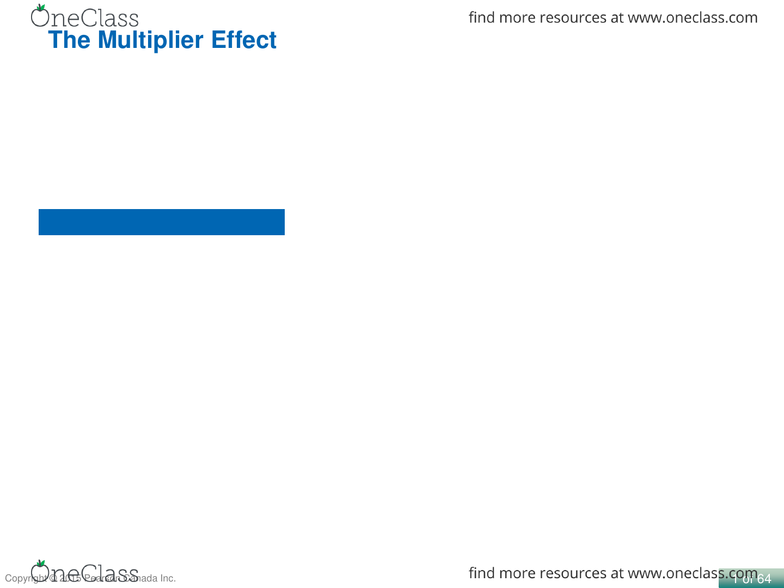Class Notes (1,100,000)
CA (620,000)
U of G (30,000)
ECON (600)
Lecture 7

# ECON 1100 - 7 - Chapter 8 - part 3.pdf

Department
Economics
Course Code
ECON 1100
Professor
Kosemple
Lecture
7

Page:
of 201 of 64
Describe the multiplier effect and use the multiplier formula to calculate changes
in equilibrium GDP.
8.4 LEARNING OBJECTIVE
The Multiplier Effect
2 of 64
Showing a Recession on the 45°-Line Diagram
Macroeconomic equilibrium can occur at any point on the 45°line.
Ideally, we would like equilibrium to occur at potential GDP.
At potential GDP, firms will be operating at their normal level of capacity, and
the economy will be at the natural rate of unemployment.
At the natural rate of unemployment, the economy will be at full employment:
Everyone in the labor force who wants a job will have one, except the
structurally and frictionally unemployed.
For equilibrium to occur at the level of potential GDP, planned aggregate
expenditure must be high enough.
3 of 64
Figure 8.11
Showing a Recession
on the 45°-Line Diagram
When the aggregate
expenditure line intersects
the 45°line at a level of
GDP below potential GDP,
the economy is in recession.
The figure shows that
potential GDP is \$2 trillion,
but because planned
aggregate expenditure is
too low, the equilibrium level
of GDP is only \$1.6 trillion,
where the AE line intersects
the 45°line.
As a result, some firms will be
operating below their normal
capacity, and unemployment
will be above the natural rate
of unemployment.
We can measure the shortfall in planned aggregate expenditure as the vertical distance
between the AE line and the 45°line at the level of potential GDP.
Means that the level of spending is short of what the economy could actually
produced. There there has to be an unemployment rate that is larger than the
natural unemployment rate = cyclic unemployment. !
This is a demand side problem. After intersect, demand is less than supply, so
we need to pick up demand. !
The better of the shortages to have (no structural issues to economy), basically
just need to get spending up. Looking at policy tools. !
!
Supply side is more difficult, output is low and you are not capable to increase
output. Longer to fix since you are increasing productivity = RandD, investment.
Need to do something to
shift the line upwards (+
shift), then it would pass
through the Y=A line at the
potential output level.